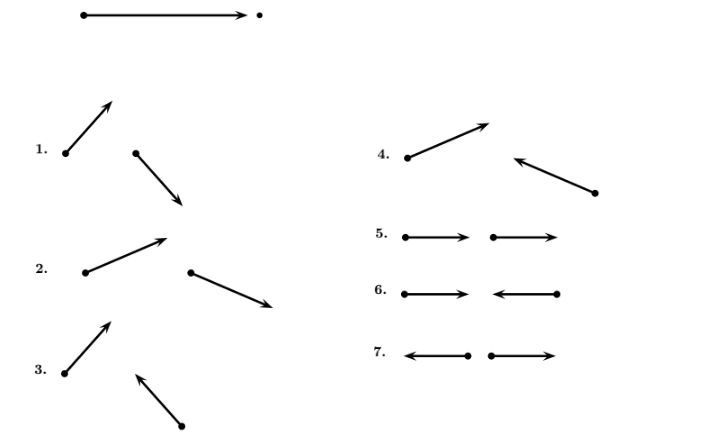# Problem: An object with an initial momentum represented by the vector below, strikes an object that is initially at rest. Which of the following sets of vectors may represent the momenta of the two objects after the collision? Note carefully: The original vector below and the following vectors are ALL DRAWN TO THE SAME LENGTH SCALE.

###### FREE Expert Solution
95% (223 ratings)
###### Problem Details

An object with an initial momentum represented by the vector below, strikes an object that is initially at rest. Which of the following sets of vectors may represent the momenta of the two objects after the collision? Note carefully: The original vector below and the following vectors are ALL DRAWN TO THE SAME LENGTH SCALE.Frequently Asked Questions

What scientific concept do you need to know in order to solve this problem?

Our tutors have indicated that to solve this problem you will need to apply the 2D Collisions concept. You can view video lessons to learn 2D Collisions. Or if you need more 2D Collisions practice, you can also practice 2D Collisions practice problems.

How long does this problem take to solve?

Our expert Physics tutor, Jeffery took 2 minutes and 35 seconds to solve this problem. You can follow their steps in the video explanation above.

What professor is this problem relevant for?

Based on our data, we think this problem is relevant for Professor Perry's class at TEXAS.